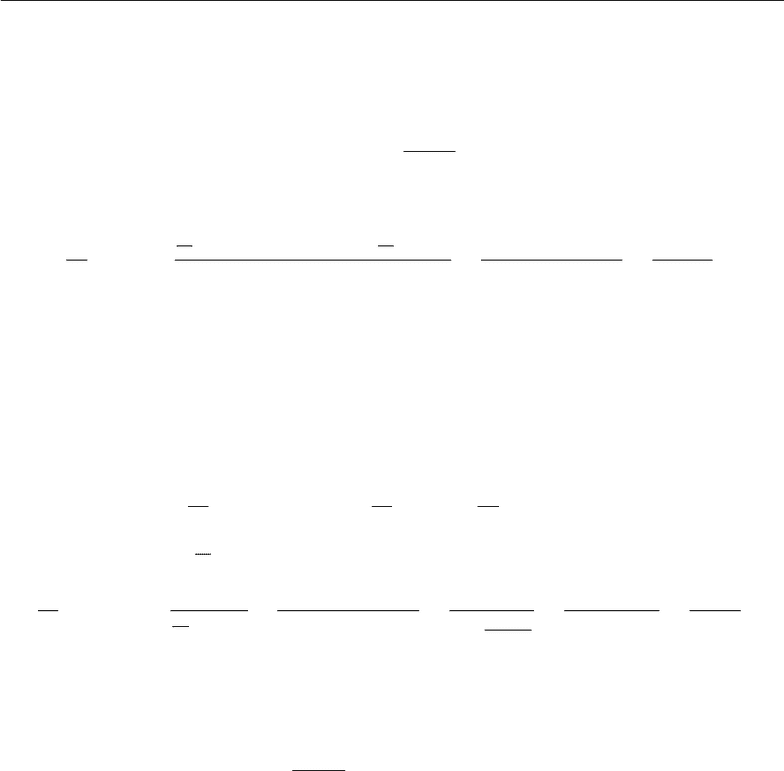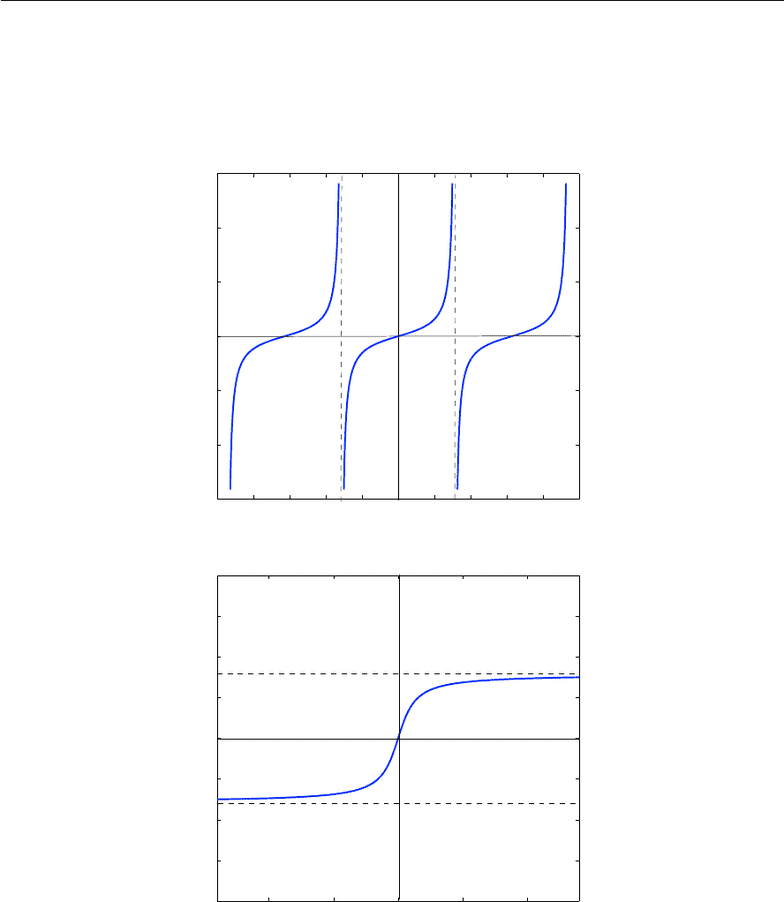Class Notes (1,100,000)
CA (620,000)
UOttawa (30,000)
MAT (1,000)
Lecture 2

# MAT1332 Lecture 2: CourseNotes2

Department
Mathematics
Course Code
MAT 1332
Professor
Robert Smith
Lecture
2

This preview shows pages 1-3. to view the full 103 pages of the document.
MAT 1332: Calculus for Life Sciences
A course based on the book
Modeling the dynamics of life
by F.R. Adler
Supplementary material
University of Ottawa
Frithjof Lutscher, Jing Li and Robert Smith?
April 13, 2010

Only pages 1-3 are available for preview. Some parts have been intentionally blurred.MAT 1332: Additional Course Notes 1
The inverse tangent function
The tangent function is deﬁned as
tan(x) = sin(x)
cos(x),
and its derivative can be compute by the quotient rule as
d
dx tan(x) =
d
dx sin(x) cos(x)sin(x)d
dx cos(x)
cos2(x)=cos2(x) + sin2(x)
cos2(x)=1
cos2(x).
In particular, the function is deﬁned for all xthat are not odd multiples of π, and the function
is monotone increasing, see Figure 1.
The inverse of the tangent is denoted as arctan or tan1and it is deﬁned in the usual way
as
arctan(tan(x)) = x, tan(arctan(x)) = x.
See Figure 1. What is its derivative? We diﬀerentiate the ﬁrst equality above by the chain
rule and ﬁnd d
dx[arctan(tan(x))] = d
dy arctan(y)d
dx tan(x)=1,
with y= tan(x), since d
dx x= 1.Hence, we can divide
d
dy arctan(y) = 1
d
dx tan(x)=cos2(x)
cos2(x) + sin2(x)=1
1 + sin2(x)
cos2(x)
=1
1 + tan2(x)=1
1 + y2.
Application to integration
We can now integrate the derivative of the arctan function to get
Z1
1 + x2dx = arctan(x) + C.
1

Only pages 1-3 are available for preview. Some parts have been intentionally blurred.MAT 1332: Additional Course Notes 2
MAT 1332: Frithjof Lutscher 2
!5!4!3!2!1012345
!15
!10
!5
0
5
10
15
x
y
y=tan(x)
x=!!/2
x=!/2
!10 !5 0 5 10
!4
!3
!2
!1
0
1
2
3
4
x
y
y=arctan(x)
y=!/2
y=!!/2
Figure 1: Graphs of the tangent function and its inverse, the arctangent function
2
Figure 1: Graphs of the tangent function and its inverse, the arctangent function
2
###### You're Reading a Preview

Unlock to view full version